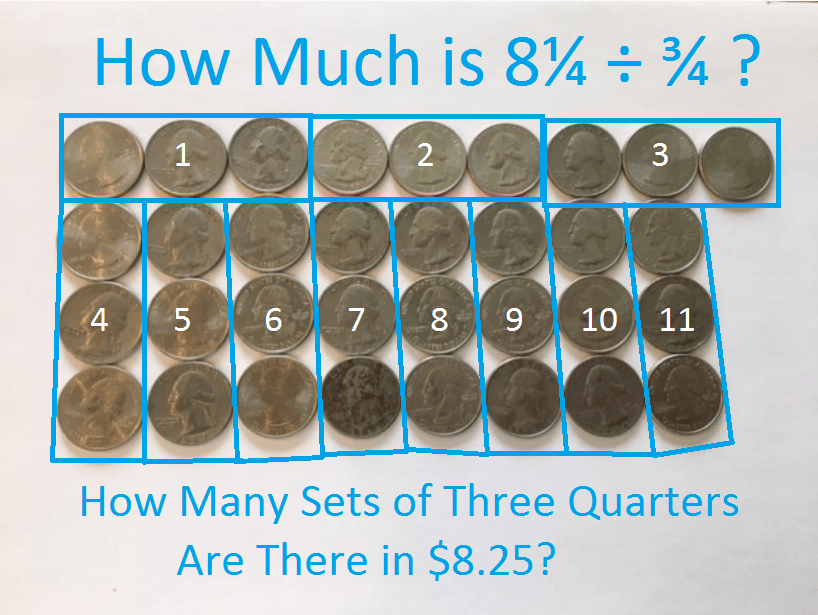# 825 Quarters Make Dividing by 25 Easy

Numbers ending in 00, 25, 50, and 75 can be divided evenly by 25. How much is 825 divided by 25? That quotient is the same as the answer to “how many quarters are in \$8.25?” (A quarter is ¼ of a dollar and is written .25 or 25¢.)You probably could visualize the answer in your head even if I hadn’t included a picture! That’s why I often ask kids the how-many-quarters question when they are stumped dividing something by 25 . It seems that kids are always able to give the quotient after that question. Notice that “8.25 ÷ .25 =” and  “8 ¼ ÷ ¼ =” have the same answer, too. You can also ask that how-many-quarters question to find the answer when something is divided by .25 or ¼.

It would almost be as easy to divide \$8.26 or \$8.39 by 25. The quotient would be the same as the problem above but with some loose change becoming the remainder. Using money for division problems could even help kids better understand dividends, divisors, quotients, and remainders.

Here’s an example of a how-many-quarters type question that will help you divide by 75, .75 or ¾.We can count and see that there are 11 sets of 3 quarters in \$8.25. That means that \$8.25 ÷ .75 is 11. It also means that 8¼ ÷ ¾ = 11.

Dividing by fractions can be a very abstract concept for students, and they may ask questions like, “What does 8¼ ÷ 1¼ even mean?” Again quarters come to the rescue! 5 quarters can be so much more friendly than 1¼ is. Shorthand for 5 quarters is the fraction, 5/4. Since they have the same denominators, dividing 8¼ by 1¼ is the same as dividing 33 by 5:Kids think money is more fun than math, but money is just a subset of mathematics which is full of lots of other fun topics, too. Here are a couple of ways other educators have used money to teach a math topic.

Jen of Beyond Tradit’l Math shared her way to teach subtracting decimals using money. Her method will surely captivate any child who tries it and even make regrouping fun:

Robert Kaplinsky uses several very short videos to keep students engaged without them actually touching any money. Check out the replies, too. Paula Beardell Krieg’s excellent \$1.00 art project is there, and that would be fun for anyone 2nd grade or older to do:

Now back to the number 825.

Clearly 825 has to be divisible by both 5 AND 3 in order for (821, 823, 827, 829) to be the fourth prime decade, which it is.

• The last digit of 825 is 5, so it is divisible by 5.
• 8 + 2 + 5 = 15, a multiple of 3, so 825 is divisible by 3.
• 8 – 2 + 5 = 11, so 825 is divisible by 11.

All numbers ending in 00, 25, 50, or 75 can have their square roots simplified. If you were trying to simplify √825, you could visualize quarters in your mind to easily divide 825 by 25. Then √825 = (√25)(√33) = 5√33

Here is 825’s factoring information:

• 825 is a composite number.
• Prime factorization: 825 = 3 × 5 × 5 × 11, which can be written 825 = 3 × 5² × 11
• The exponents in the prime factorization are 1, 2, and 1. Adding one to each and multiplying we get (1 + 1)(2 + 1)(1 + 1) = 2 × 3 × 2 = 12. Therefore 825 has exactly 12 factors.
• Factors of 825: 1, 3, 5, 11, 15, 25, 33, 55, 75, 165, 275, 825
• Factor pairs: 825 = 1 × 825, 3 × 275, 5 × 165, 11 × 75, 15 × 55, or 25 × 33
• Taking the factor pair with the largest square number factor, we get √825 = (√25)(√33) = 5√33 ≈ 28.72281